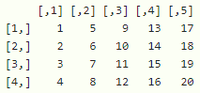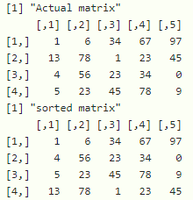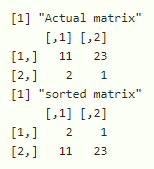GFG App
Open AppBrowser
Continue

# Sort Matrix According to First Column in R

In this article, we will discuss how to sort a matrix according to the first column in R programming language.

Matrix in use:We can sort the matrix according to a specific column by using the order() function with indexing. To sort according to first column 1 is passed in place of column index.

Syntax:

matrix[order(matrix[ , 1]), ]

where,

• matrix is the input matrix pass with an index
• order() function takes one parameter that is matrix with 1 st column index

Example: R program to sort a matrix according to first column

## R

 `# create a matrix with 4 rows and 5 columns  ` `# - 20 elements ` `data = ``matrix``(``c``(1, 13, 4, 5, 6, 78, 56, 23, 34, 1, 23,  ` `                ``45, 67, 23, 34, 78, 97, 45, 0, 9), ` `              ``nrow=4, ncol=5) ` ` `  `print``(``"Actual matrix"``) ` ` `  `print``(data) ` ` `  `print``(``"sorted matrix"``) ` ` `  `# display sorted data according to first column ` `final = data[``order``(data[, 1]), ] ` ` `  `print``(final) `

Output:Example: R program to sort a matrix according to first column

## R

 `# create a matrix with 2 rows and 2 columns ` `# - 4 elements ` `data= ``matrix``(``c``(11,2,23,1),nrow=2,ncol=2) ` ` `  `print``(``"Actual matrix"``) ` ` `  `print``(data) ` ` `  `print``(``"sorted matrix"``) ` `  `  `# display sorted data according to first column ` `final=data[``order``(data[ , 1]), ] ` ` `  `print``( final)`

Output:My Personal Notes arrow_drop_up